# Permutations, Math Formulas For GMAT Notes | Study Quantitative Aptitude for GMAT - GMAT

## GMAT: Permutations, Math Formulas For GMAT Notes | Study Quantitative Aptitude for GMAT - GMAT

The document Permutations, Math Formulas For GMAT Notes | Study Quantitative Aptitude for GMAT - GMAT is a part of the GMAT Course Quantitative Aptitude for GMAT.
All you need of GMAT at this link: GMAT

MATH FORMULAS

Permutations:

Note: Multiple events means multiply! This rule applies to ALL permutation, combination and probability questions in some way.

No math formula required. Draw a picture, keep track of the results and multiply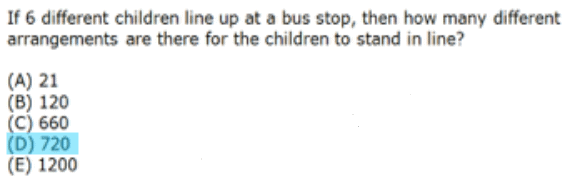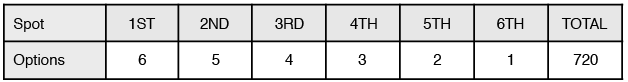Combinations:

Combination Formula

= Number of items
= Number of items to be selected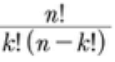Example: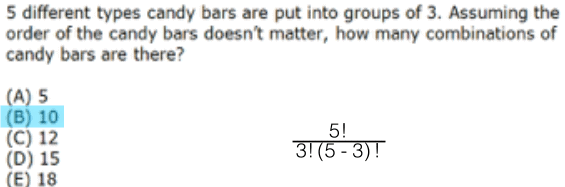Probability:

Probability =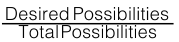“and” = Multiply

Example: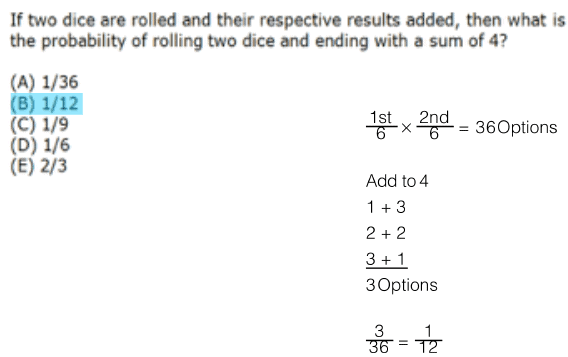The document Permutations, Math Formulas For GMAT Notes | Study Quantitative Aptitude for GMAT - GMAT is a part of the GMAT Course Quantitative Aptitude for GMAT.
All you need of GMAT at this link: GMATUse Code STAYHOME200 and get INR 200 additional OFF

## Quantitative Aptitude for GMAT

75 videos|87 docs|156 tests

Track your progress, build streaks, highlight & save important lessons and more!

,

,

,

,

,

,

,

,

,

,

,

,

,

,

,

,

,

,

,

,

,

,

,

,

;